# Helmholtz equation

The partial differential equationwhereis a constant. The Helmholtz equation is used in the study of stationary oscillating processes. If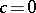, the Helmholtz equation becomes the Laplace equation. If a functionappears on the right-hand side of the Helmholtz equation, this equation is known as the inhomogeneous Helmholtz equation.

The usual boundary value problems (Dirichlet, Neumann and others) are posed for the Helmholtz equation, which is of elliptic type, in a bounded domain. A value offor which a solution of the homogeneous Helmholtz equation not identically equal to zero and satisfying the corresponding homogeneous boundary condition exists, is called an eigen value of the Laplace operator (of the corresponding boundary value problem). In particular, for the Dirichlet problem all eigen values are positive, and for the Neumann problem they are all non-negative. It is known that a solution of the boundary value problem for the Helmholtz equation is not unique for a value ofwhich coincides with an eigen value. If, on the other hand, the value ofis not an eigen value, the uniqueness theorem is valid.

Boundary value problems for the Helmholtz equation are solved by the ordinary methods of the theory of elliptic equations (reduction to an integral equation, variational methods, methods of finite differences).

In the case of an unbounded domain with compact boundary one can state exterior boundary value problems for the Helmholtz equation; if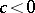, these have a unique solution which tends to zero at infinity. If, solutions tending to zero at infinity are usually not unique. In such cases additional restrictions are imposed to obtain a unique solution (cf. Exterior and interior boundary value problems; Limit-absorption principle).

The following mean-value formula is valid for a solution of the Helmholtz equation which is regular in a domain: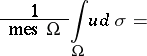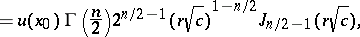whereis the sphere of radiuswith centre at a point, which must lie entirely within, and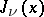is the Bessel function of order(cf. Bessel functions).

The equation was studied by H. Helmholtz in 1860, who obtained the first theorems on the solution of boundary value problems for this equation.